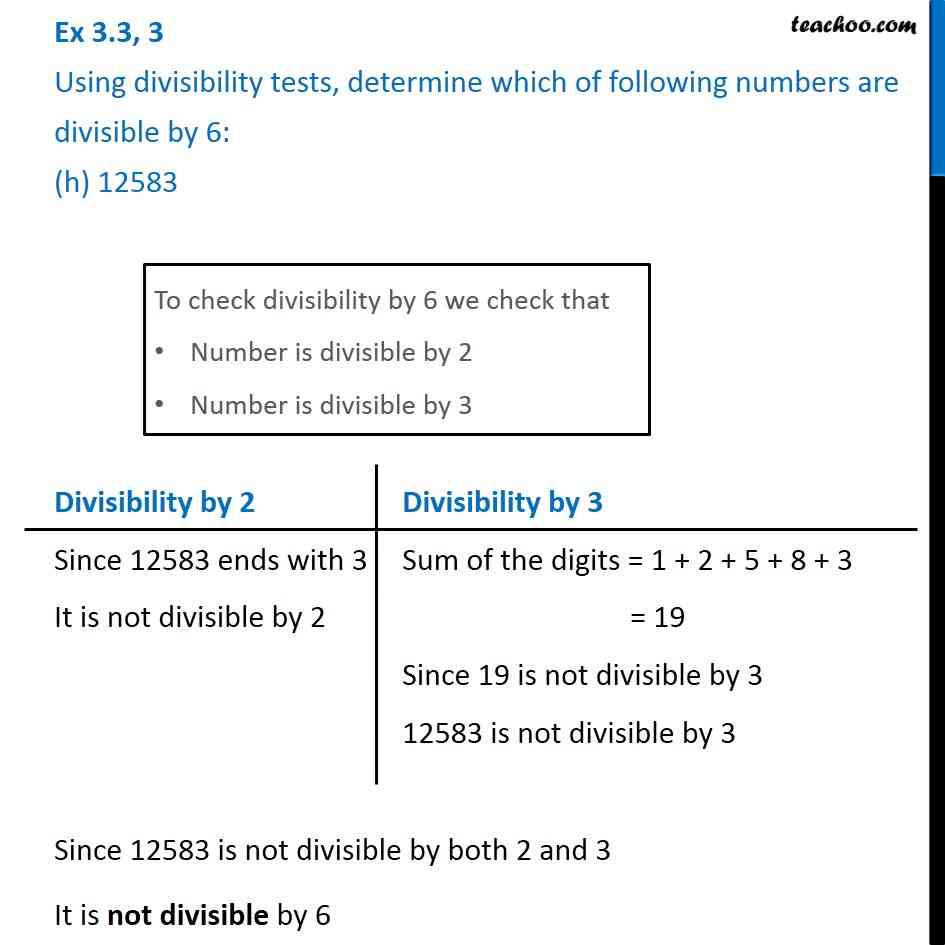Ex 3.3

Chapter 3 Class 6 Playing with Numbers
Serial order wiseLearn in your speed, with individual attention - Teachoo Maths 1-on-1 Class

### Transcript

Ex 3.3, 3 Using divisibility tests, determine which of following numbers are divisible by 6: (h) 12583To check divisibility by 6 we check that Number is divisible by 2 Number is divisible by 3 Divisibility by 2 Since 12583 ends with 3 It is not divisible by 2 Divisibility by 3 Sum of the digits = 1 + 2 + 5 + 8 + 3 = 19 Since 19 is not divisible by 3 12583 is not divisible by 3 Since 12583 is not divisible by both 2 and 3 It is not divisible by 6